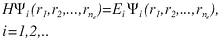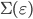#### Electronic Structure Theory

Electronic structure is a terminology widely used in chemistry and solid state physics to describe the distribution of electrons in molecules, solids, and other nanoscale objects. Because electrons serve as the quantum glue that binds atoms into molecules or solids, understanding their distribution and the change in distribution is the key to modern materials design, analysis, and engineering.

In this project, we are developing mathematical analysis, numerical algorithms and software tools to enable scientists at the Molecular Foundry and Advanced Light Source to study both the ground- and excited-state electronic structure of complex materials and interfaces relevant for energy research.  These tools can be used, for example, to interpret photoemission spectroscopy experimental results and to predict properties of potential materials that are suitable for photovoltaic cells and batteries.

Early in the twentieth century, it was shown that the electronic structure of an atomistic system could be determined from the solution of a quantum many-body problem described by the Schrodinger equation

where                                                 is a many-body wavefunction; the differential operator  H is a many-body Hamiltonian that describes the kinetic energy and the Coulomb interaction between electron-electron and electron-nucleus pairs; and       is the total energy level of the system.

However solving the Schrodinger's equations directly is not computationally feasible except for systems with one or two atoms, due to the exponential increase in the numbers of degrees of freedom with respect to the number of atoms.

One way to get around this difficulty is to use density functional theory (DFT) to express the total energy of an electronic system in terms of a functional of the electron density. In particular, the ground state properties of a many-electron system can be obtained by solving a nonlinear eigenvalue problem known as the Kohn-Sham equatio
where  H  is the Kohn-Sham Hamiltonian that depends on the electron density                       with orthogonality condition

Although, the Kohn-Sham eigenvalue problem is defined in 3D, solving this problem efficiently is still challenging for large systems with tens of thousands of atoms. The complexity of the exisiting algorithm is O(N^3) , where N is the number of atoms in the system under study.

Our work reduces the computational cost of the ground state calculation through several key steps:

• Reduce the complexity of electron density evaluation.  The existing algorithms has O(N^3)  complexity. Our approach, which is based on expressing the electron density as a linear combination of the diagonal of the inverses of a number of shifted Kohn-Sham Hamiltonians,

• This method is called the pole expansion and selected inversion method (PEXSI).  The PEXSI method is applicable to both metallic and insulating systems, and reduces the complexity to at most  O(N^2) for systems of large size.  The PEXSI method is also massively parallelizable and scales to 10,000~100,000 processors.

• Reduce the prefactor of the electron density evaluation through novel discretization of the Kohn-Sham equation. We are currently developing the Discontinuous Galerkin (DG) based method for solving the Kohn-Sham density functional theory: DGDFT. The key of this method is a new basis set called the adaptive local basis set (ALB), which is constructed by solving Kohn-Sham problems locally in the real space.  The ALB automatically and systematically builds the rapidoscillations of the Kohn-Sham orbitals around the nuclei as well as environmental effects into the basis functions.  Each basis function is discontinuous in the global domain.  The continuous Kohn-Sham orbitals and the electron density are evaluated from the discontinuous basis functions using the discontinuous Galerkin (DG) framework, which provides full flexibility for constructing basis set.

• Accelerate the convergence of nonlinear eigensolver by developing effective preconditioners. For large scale metallic systems, the number of iterations can increase rapidly with the system size and makes the large scale electronic structure calculation prohibitively expensive. We developed an efficient preconditioner for accelerating the SCF iteration for solving the KSDFT for large scale inhomogeneous metallic systems, which is called the elliptic preconditioner.  The elliptic preconditioner solves an elliptic equation, which can be derived from a piecewise constant approximation to the polarizability matrix of the system. Such elliptic equation can be efficiently solved.

The ground-state electronic structure calculation is the starting point for excited-state calculation. One way to study the simplest type of excitation, i.e. single electron excitation, is to examine the probability amplitude of finding an electron at position r_1 and time , t_1, when an electron is added or removed from position r_2 at time  t_2.  It can be shown that this probability amplitude, denoted by G(r_1,t_1,r_2,t_2), is the Green's function of a special type of Hamiltonian operator in the form of                         , where        is a single electron Hamiltonian for an non-interacting electron, and         , which is called the self-energy, describes the response of an electron to the external perturbation induced by itself.  If we take the Fourier transform of  G  with respect to t_2-t_1 , we obtain a frequency-domain representation of the Green's function                     . What is important about this representation is that the poles of                           give the single-electron excitation energies, i.e., energies required to remove or insert an electron. It is these energy levels that ultimately determine the likelihood of finding an electron at one place and time when an electron is added or removed at another place and time.  To some extent, they give the signature of a material (i.e., the band structure) and indicate how well it will perform in electronic or optical devices.

Since the Green's function is associated with  H, finding these the poles of the Green's function is equivalent to computing eigenvalues of H. Therefore, in a computational procedure, we solve what is called a Dyson's equation

where        is called quasi-particle energy, which corresponds to a pole in  G, and         is a quasi-particle orbital or wavefunction.   The quasi-particle equation is a nonlinear eigenvalue problem because the self-energy       is a function of the eigenvalue to be computed.

The difficulty of this problem lies in the fact that

• the exact form of               is unknown, and must be approximated

• the existing approximation is costly to compute

• the nonlinear structure of the eigenvalue problem is largely unknown, convergence of the existing algorithm is not guaranteed.

In our project, we will try to address the above issues through analysis and algorithm development.

We will begin from one commonly used self-energy approximation, which expresses           as the product of the unknown Green's function G and a screened Coulomb interaction, often denoted by W.  This approach is known as the GW approximation.

The screening of the Coulomb interaction which account for the response of the many-electron system to external perturbation is described by a dielectric operator           , which is also energy (frequency) dependent.

A naive way to calculate            requires all eigenvectors of the ground state Kohn-Sham Hamiltonian to be computed. This is extremely costly.  We are currently pursuing an alternative approach that only requires a fraction of the eigenvalues and eigenvectors.

Another important computational issue in the GW approximation is the evaluation of the self-energy at multiple frequencies.  Each evaluation requires performing a numerical integration of a nearly singular integrand. We are developing a special integration scheme that requires few quadrature points without sacrificing the accuracy of the integral.

Once the self-energy calculation becomes sufficiently fast, we can then analyze the convergence of iterative algorithms for solving the Dyson's equations, develop acceleration techniques, and investigate other types of approximations to the self-energy.